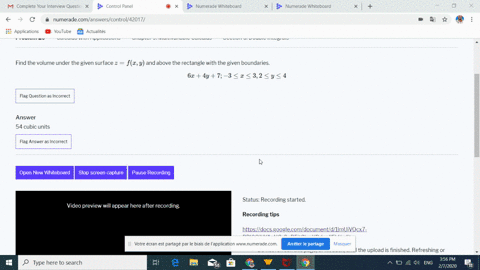Enroll in one of our FREE online STEM bootcamps. Join today and start acing your classes!View Bootcamps## Discussion

You must be signed in to discuss.

## Video Transcript

Okay, So another integral here. Double integral. All right, Andy, it's so why's going from one, two three Xs going from one two. You know, Grant is X squared. Need to the execute plus two. Why? T x Hey. Bye. Okay, so we'LL take an anti derivative with respect to X first. And if we look here we take a derivative of this We'LL get something involving x squared So let you be x cubed Plus two Why differentiate with respect x three X squared x Or, in other words, X squared DX is one third Did you case? It's a nice substitution. So we have one, two, three and then now for you See, that's going to be one must tio It's gonna be a pal's tio. When? Then this is just gonna b e to the u quick and then we have a factor. Ves Well, one third with substitution. Do you know why? So leave this one third out We have the inner girl. One, two, three Ah, anti derivative of any to you. Interesting to you. So I just need to evaluate cave's too. Why arness e to the one plus two i why and now here the anti derivative of easy exposed Teo I and limbless too. Why all involved? Just same thing, but dividing by two. So if we divide by two, get one six and then we have need to the A plus two Why minus e to the one was too. Why evaluated from one to three and that'LL be one six. So we have e to the six. Fourteen minus e to the one six seven minus see minus the second in points that need to the tables to ten and then minus minus. Plus I e to the one was too.